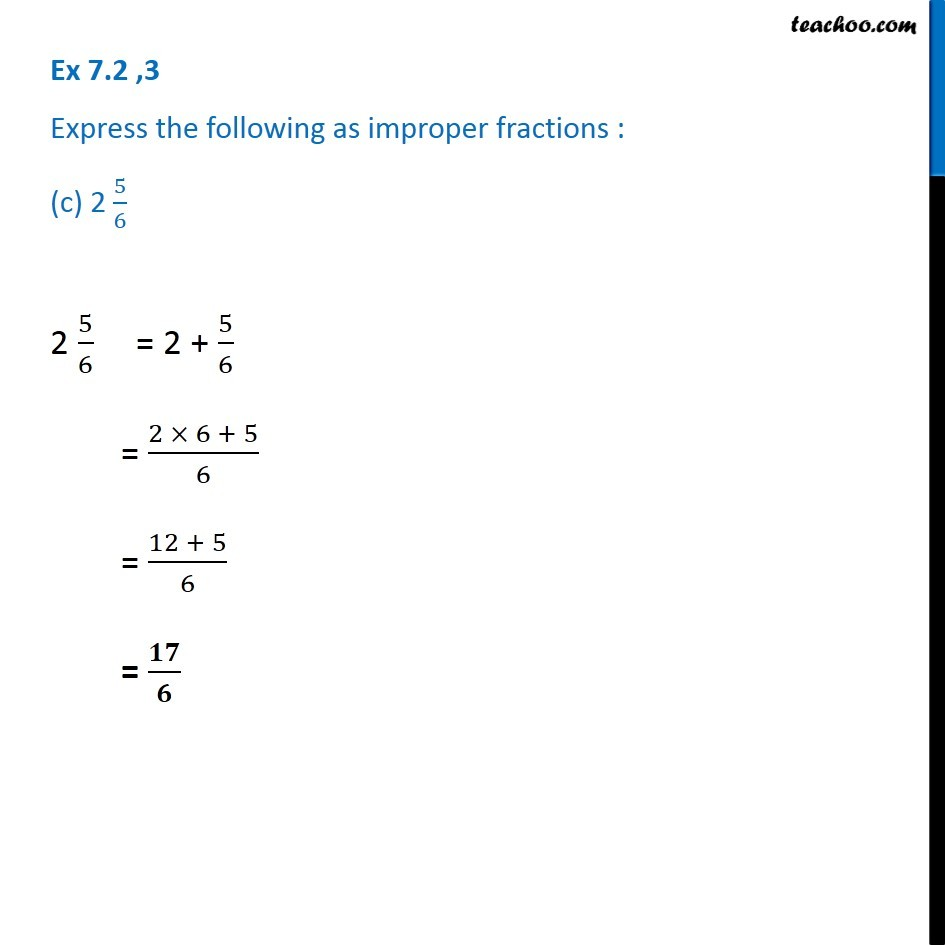1. Chapter 7 Class 6 Fractions
2. Serial order wise
3. Ex 7.2

Transcript

Ex 7.2 ,3 Express the following as improper fractions : (c) 2 5/6 2 5/6 = 2 + 5/6 = (2 × 6 + 5)/6 = (12 + 5)/6 = 𝟏𝟕/𝟔